Kirchoff's laws / RC Circuits Examples

Example #1

Problem:

Find the currents through all the resistors in the circuit below: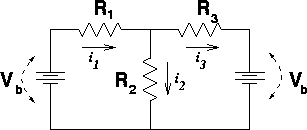DATA: Vb = 12 V, R1 = 10 W, R2 = 15 W, R3 = 20 W

Solution:

Summing the voltages around the left and right loops gives the following two equations:

1.2.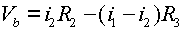where i3 has been replaced by i1 - i2. Multiplying Eq. (1) by R3, multiplying Eq. (2) by R1, then adding the equations yields: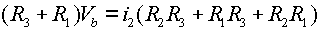which rearranged yields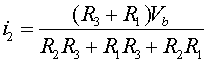Once i2 is known, Eq. (1) can be used to get i1, and i3 can be found as the difference i1 - i2.

i2 = 0.554 amps, i1= .369 amps, i3 = -.185 amps

Example #2

Problem:

Find the charges on all the capacitors in the circuit below: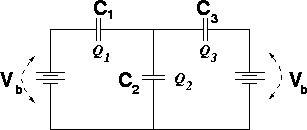DATA: Vb = 12 V, C1 = 10 mF, C2 = 15 mF, C3 = 20 mF

Solution:

Summing the voltages around the left and right loops gives the following two equations

1.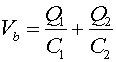2.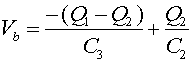where Q3 has been replaced by Q1 - Q2. Dividing Eq. (1) by C3, dividiing Eq. (2) by C1, then adding the equations yields: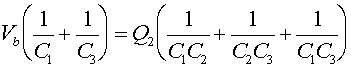which rearranged yields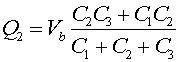Once Q2 is known, Eq. (1) can be used to get Q1, and Q3 can be found as the difference Q1 - Q2.

Q2 = 120.0 mC, Q1= 40.0 mC, Q3 = -80 mC

Example #3

The circuit below has been in position a for a long time. At time t = 0 the switch is thrown to position b. DATA: Vb = 12 V, C = 10 mF, R = 20 W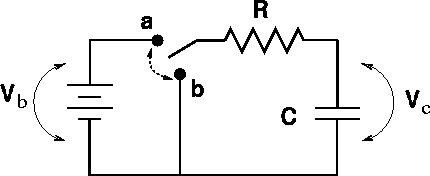a.) What is the curnent through the resistor just BEFORE the switch is thrown?

I = 0

b.) What is the current through the resistor just AFTER the switch is thrown?

Solution: I = V/R

I = 0.6 amps

c.) What is the charge across the capacitor just BEFORE the switch is thrown?

Solution: Q = CV

Q = 120 mC

d.) What is the charge on the capacitor just AFTER the switch is thrown?

Solution: Charge does not change instantaneously.

Q = 120 mC

e.) What is the charge on the capacitor at at time t = 0.3 msec after the switch is thrown?

Solution: Q = Q0exp(-t/t) , where t = RC = 0.2 msec

Q = 26.8 mC

Example #4

Considering the same circuit, only with the switch thrown from b to a at time t = 0 after having been in position b for a long time. DATA: Vb = 12 V, C = 10 mF, R = 20 Wa.) What is the curnent through the resistor just BEFORE the switch is thrown?

I = 0

b.) What is the current through the resistor just AFTER the switch is thrown?

Solution: I = V/R

I = 0.6 amps

c.) What is the charge across the capacitor just BEFORE the switch is thrown?

Solution: Q = CV

Q = 0

d.) What is the charge on the capacitor just AFTER the switch is thrown?

Solution: Charge does not change instantaneously.

Q = 0

e.) What is the charge on the capacitor at at time t = 0.3 msec after the switch is thrown?

Solution: Q = Q0(1.0 - exp(-t/t)) , where t = RC = 0.2 msec

Q = 93.2 mC

Kirchoff's laws / RC Circuit's index